# Scientific Notation From Scientific to Standard Notation Multiplying

• Slides: 10Scientific Notation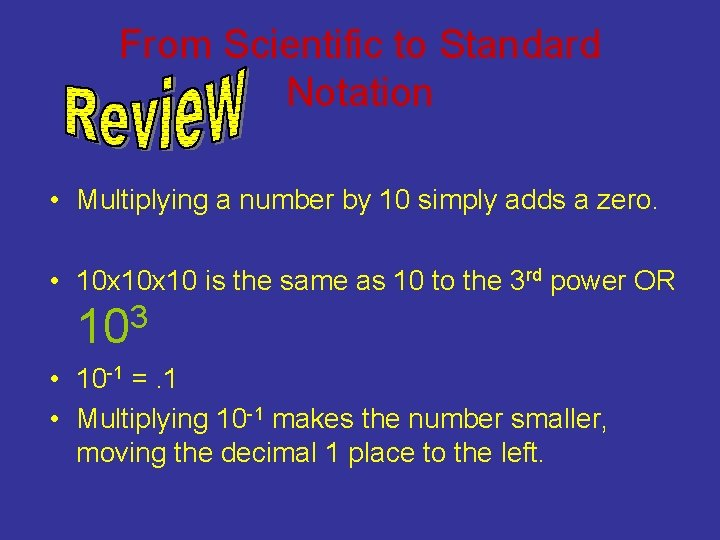From Scientific to Standard Notation • Multiplying a number by 10 simply adds a zero. • 10 x 10 is the same as 10 to the 3 rd power OR 103 • 10 -1 =. 1 • Multiplying 10 -1 makes the number smaller, moving the decimal 1 place to the left.Definition!!! A number written in Scientific notation is a Number between 1 and 10 Multiplied by a power of ten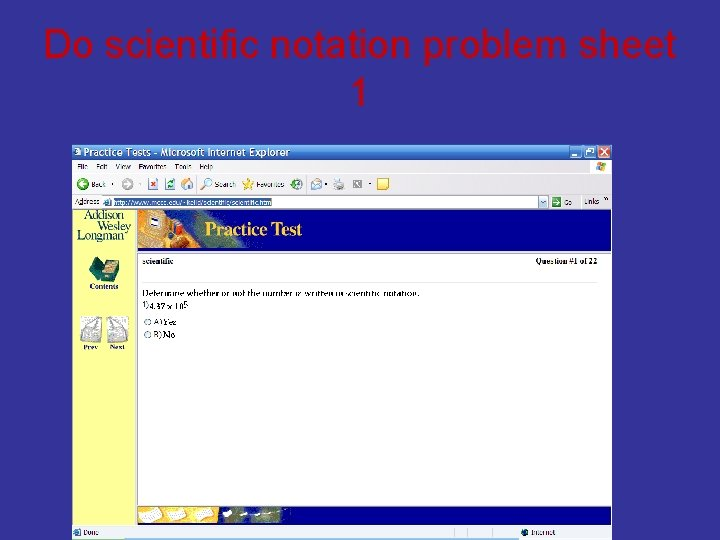Do scientific notation problem sheet 1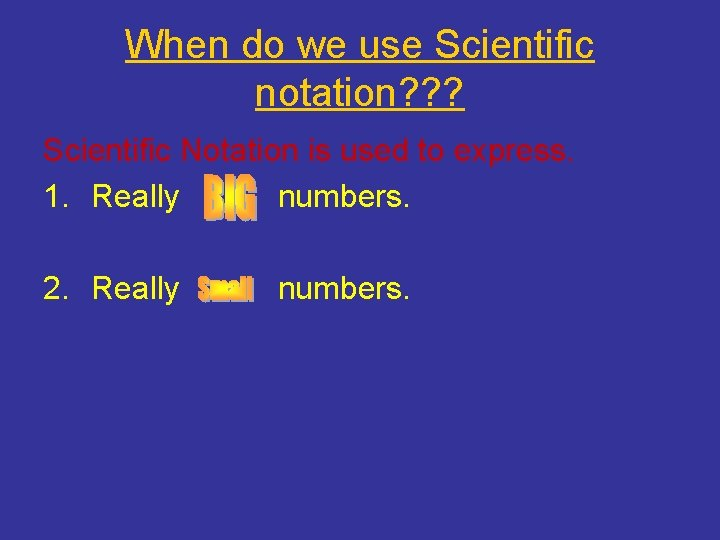When do we use Scientific notation? ? ? Scientific Notation is used to express. 1. Really numbers. 2. Really numbers.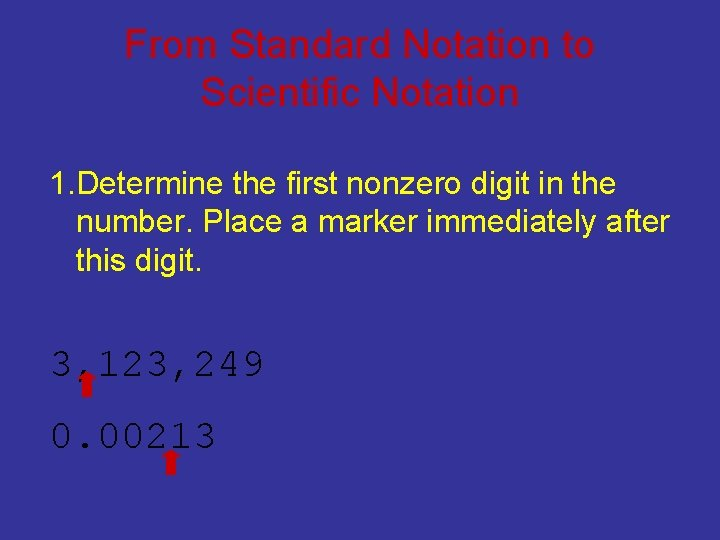From Standard Notation to Scientific Notation 1. Determine the first nonzero digit in the number. Place a marker immediately after this digit. 3, 123, 249 0. 00213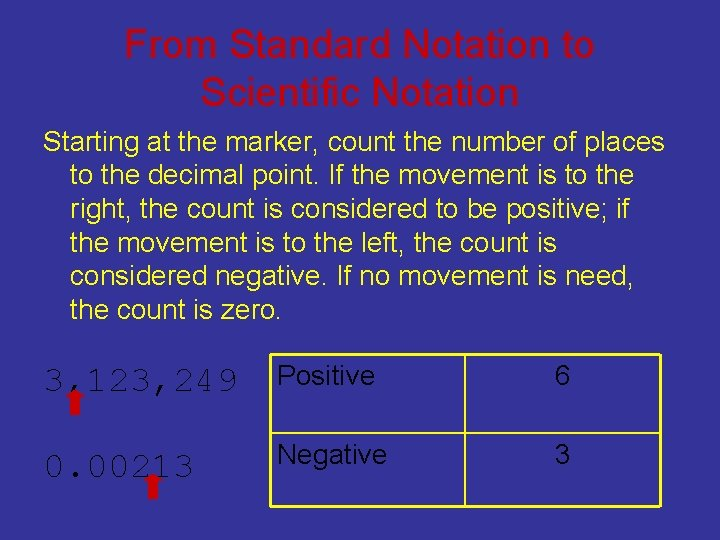From Standard Notation to Scientific Notation Starting at the marker, count the number of places to the decimal point. If the movement is to the right, the count is considered to be positive; if the movement is to the left, the count is considered negative. If no movement is need, the count is zero. 3, 123, 249 Positive 6 0. 00213 Negative 3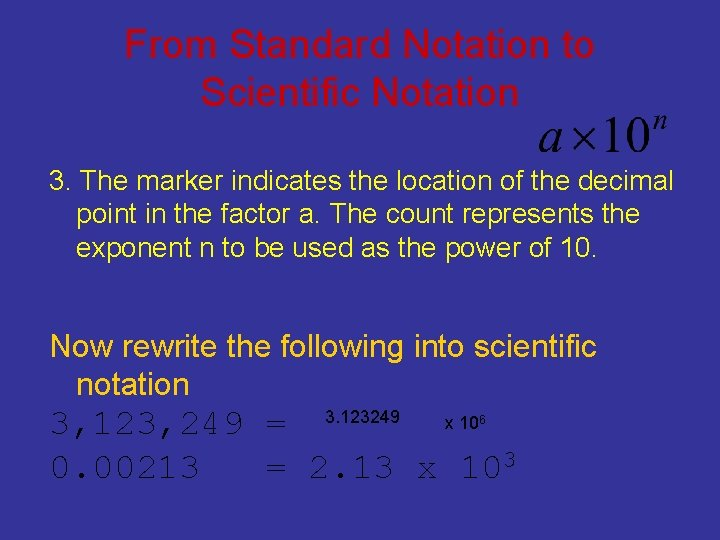From Standard Notation to Scientific Notation 3. The marker indicates the location of the decimal point in the factor a. The count represents the exponent n to be used as the power of 10. Now rewrite the following into scientific notation 3, 123, 249 = 3. 123249 x 10 0. 00213 = 2. 13 x 103 6To convert from scientific notation to standard notation 1. Identify the exponent. +/2. 123 x 103 1. 23 x 10 -4 numberTo convert from scientific notation to standard notation If the exponent on 10 is positive ("+"), move the decimal to the right the same number of places as the exponent. Add zeros as necessary. If the exponents on 10 is negative("-"), move the decimal point to the left the same number of places as the exponent. Add zeros as necessary Scientific notation Standard notation 2. 123 x 103 2123. 1. 23 x 10 -4 0. 000123